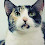## Sunday, February 25, 2007

### On NP in BQP

In the wake of a misleading Economist article on the D-Wave quantum computer, Scott argues why he believes quantum computers cannot efficiently solve NP-complete problems, i.e., that the complexity class BQP does not contain NP. Let's look at that question purely from a computational complexity viewpoint.

Ideally we would like a mathematical proof that NP ⊄ BQP. But any such proof would imply P≠NP, one of the great open problems in mathematics.

Moving on, complexity theorists give evidence that a statement Q is false by a "Pigs Can Fly" argument, by showing that Q implies something else we don't believe. For example

• If there are sparse NP-complete sets then P=NP.
• If NP has efficient probabilistic algorithms (NP⊆BPP), then the polynomial-time hierarchy collapses.
• If good pseudorandom generators don't exist then exponential time has small circuits.
But we don't have any known consequences of NP⊆BQP.

What we do have is the result of Bennett, Bernstein, Brassard, Vazirani that BQP can't solve black-box NP problems, a relativized separation of NP from BQP. Relativized results like this don't tell us whether or not a statement is true, just that any proof that NP in BQP would require nonrelativizing techniques. So the sentence from the Economist article

In principle, by putting a set of entangled qubits into a suitably tuned magnetic field, the optimal solution to a given NP-complete problem can be found in one shot.
does not reflect current knowledge.

Can one have a nonrelativizing proof that NP is in BQP? We do have precedent. My very first theorem gave a relativized world where co-NP does not have interactive proof systems. We published the result in a 1988 paper Are there interactive protocols for co-NP languages? and we conjectured the answer was no. We were wrong.

But so far interactive proof and related systems have been the only source of reasonable nonrelativized proof techniques that we have seen in computational complexity and we haven't had any success using this algebraic structure of arithmetized satisfiability to make efficient quantum algorithms for NP problems.

I do conjecture that NP ⊄ BQP and most computational complexity theorists would agree with me. But until we see some negative consequences of NP in BQP, computational complexity theory has so far failed to give any real evidence that NP ⊄ BQP. We believe that NP ⊄ BQP for the same reason we believe that there are no probabilistic polynomial-time algorithms for Factoring—despite considerable effort, failure to find any algorithmic techniques that might solve the problem.

1.I had a brief bit of dismay when I read that article the other week; they're usually fairly good about getting such details right.

If I'd been near a computer at the time I'd have written in a letter myself. Sadly I forgot to do so later...

Complicating things is fact that I strongly suspect that we can go as far as PSPACE "practically", just not with anything so pedestrian as a quantum computer. The full argument is too small to fit in the margin here, however...

1.2.I fear it is not the Economist who did the mistake, it is D-Wave who believes that their quantum computer can solve NP.

"Quantum-computer technology can solve what is known as “NP-complete” problems."

(Source: D-Wave News)

I am not sure whether this increases my trust in their announcements...

3."Quantum-computer technology can solve what is known as “NP-complete” problems."

Note that also classical computers can solve NP-complete problems. What they misleadingly suggest is just that this was possible efficiently on quantum computers.

4.How about consequences of BQP = QMA. We must have some of these?

5.There's also the complicating factor that D-wave CAN solve NP-complete problems efficiently, but it does so by being a massively parallel, hardware quantum annealing device--i.e. it finds good solutions to those NP-complete problems that can be stated as optimization problems, and does not solve NP in general.